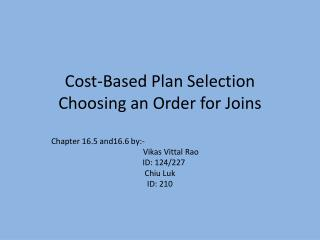DownloadDownload PresentationCost-Based Plan Selection Choosing an Order for Joins

# Cost-Based Plan Selection Choosing an Order for Joins

Download Presentation## Cost-Based Plan Selection Choosing an Order for Joins

- - - - - - - - - - - - - - - - - - - - - - - - - - - E N D - - - - - - - - - - - - - - - - - - - - - - - - - - -
##### Presentation Transcript

1. Cost-Based Plan SelectionChoosing an Order for Joins Chapter 16.5 and16.6 by:- Vikas Vittal Rao ID: 124/227 Chiu Luk ID: 210

2. Agenda • Outline • Cost Estimation • Histograms • Computation of statistics • Reducing cost of heuristics • Enumerating Physical Plans • List of other approaches

3. Outline • Query Optimizer estimates the “cost” of query evaluation. • This “cost” is based on number of disk I/O’s. • Disk I/O’s influenced by:- • Logical operators used • Size of intermediate results • The ordering of similar operations, especially joins (discussed next in 16.6)

4. Cost Estimation • Query Optimizer keeps track of certain parameters like: • T(R) – number of tuples in a relation R • V( R , a ) – number of unique values in R for attribute ‘a’ • B(R) – number of blocks in which R can fit. The Optimizer also computes a “histogram” of the above parameters.

5. Histograms • Equal Width - divide value range by fixed width w and keep counts of each width • Equal Height – similar to equal width, we pick the lowest value v0, a fraction p and keep count of values which are at “p from the lowest, 2 p from the lowest, etc” up to the highest value. • Most frequent values – list of most frequent values and the number of their occurances • Using histograms helps estimate the sizes of joins more accurately(than previously discussed methods)

6. Computation of Statistics • Statistics are fault-tolerant, good for use in estimates; e.g. small error does not significantly change out come. • Inspect the distribution of values across records in relation • Computation on entire relation is expensive; however, can be computed using smaller sample size

7. Reducing Cost by Heuristics • Applies for logical query plan • Estimate cost before and after a transformation • Only choose/apply transformation when cost estimations show beneficial • Example: • Deferring duplicate elimination is better

8. Enumerating Physical Plans • Baseline approach (exhaustive): consider all combinations, pick the smallest cost plan • Other approaches categorized into: • Top-down: compute cost from root, take the best • Bottom-up: compute cost for all combinations for a sub-expression, select the best, move up until root evaluated

9. List of Other Approaches • Heuristic selection • Branch-and-bound plan enumeration: keep record of best cost, skip to next plan if current plan exceed best known cost • Hill climbing • Dynamic programming (PP): keep least cost of each sub-expression; work bottom-up • Selinger-style optimization: improved version of DP; keep others beneficial plans besides least cost plans

10. Thank you. Chiu Luk will continue from this point.

11. Introduction • This section focuses on critical problem in cost-based optimization: • Selecting order for natural join of three or more relations • Compared to other binary operations, joins take more time and therefore need effective optimization techniques

12. Significance of Left and Right Join Arguments • The argument relations in joins determine the cost of the join • The left argument of the join is • Called the build relation • Assumed to be smaller • Stored in main-memory

13. Significance of Left and Right Join Arguments • The right argument of the join is • Called the probe relation • Read a block at a time • Its tuples are matched with those of build relation • The join algorithms which distinguish between the arguments are: • One-pass join • Nested-loop join • Index join

14. Join Trees Order of arguments is important for joining two relations Left argument, since stored in main-memory, should be smaller With two relations only two choices of join tree With more than two relations, there are n! ways to order the arguments and therefore n! join trees, where n is the no. of relations

15. Left-Deep Join Trees Consider 4 relations. Different ways to join them are as follows

16. In fig (a) all the right children are leaves. This is a left-deep tree In fig (c) all the left children are leaves. This is a right-deep tree Fig (b) is a bushy tree Considering left-deep trees is advantageous for deciding join orders

17. Dynamic Programming to Select a Join Order and Grouping • Three choices to pick an order for the join of many relations are: • Consider all of the relations • Consider a subset • Use a heuristic o pick one • Dynamic programming is used either to consider all or a subset • Construct a table of costs based on relation size • Remember only the minimum entry which will required to proceed

18. Dynamic Programming with More Detailed Cost Functions • Disadvantage of dynamic programming is that it does not involve the actual costs of the joins in the calculations • Can be improved by considering • Use disk’s I/O for evaluating cost • When computing cost of R1 join R2, since we sum cost of R1 and R2, we must also compute estimates for there sizes

19. A Greedy Algorithm for Selecting a Join Order • It is expensive to use an exhaustive method like dynamic programming • Better approach is to use a join-order heuristic for the query optimization • Greedy algorithm is an example of that • Make one decision at a time and never backtrack on the decisions once made

20. Thank you